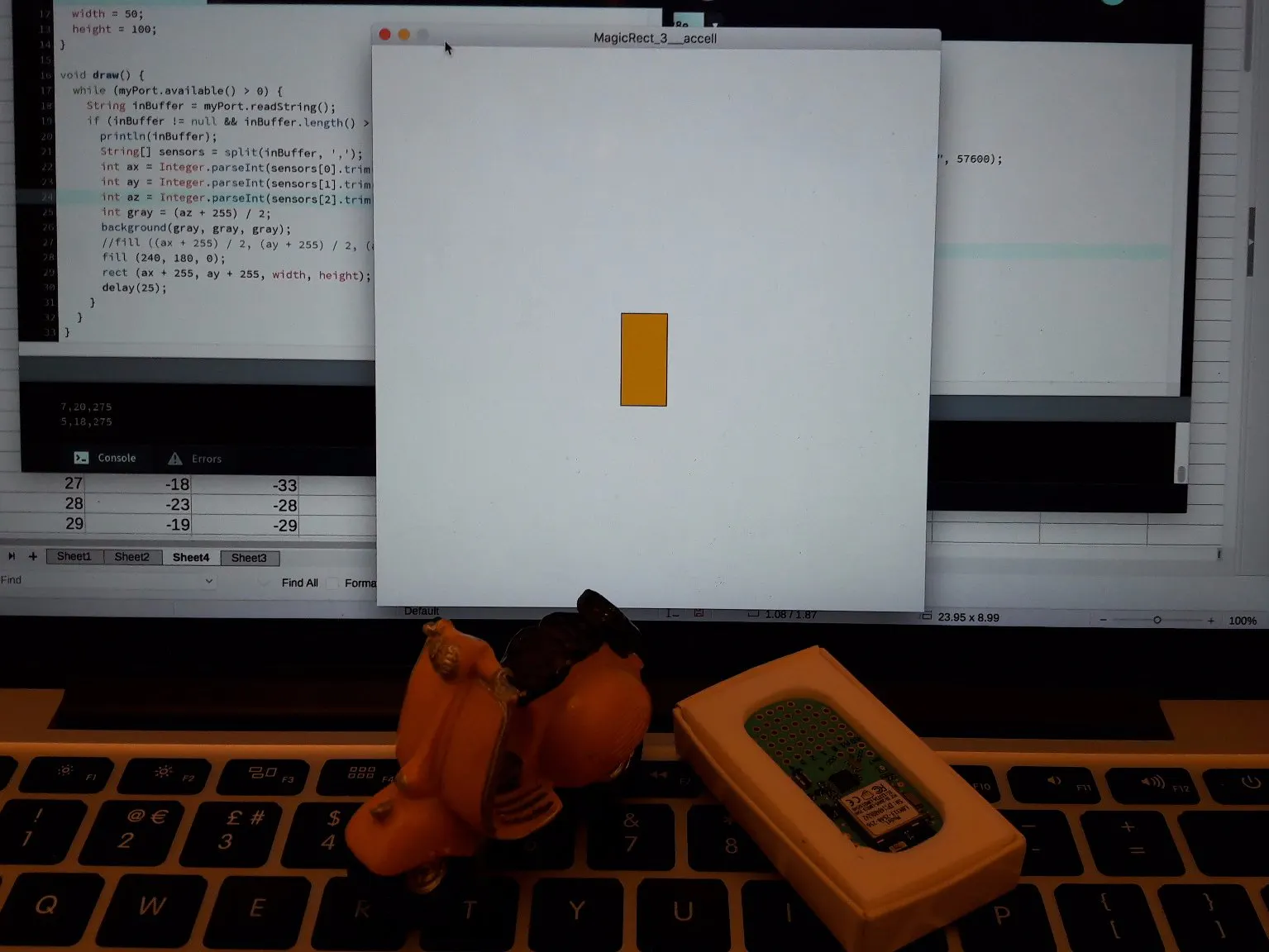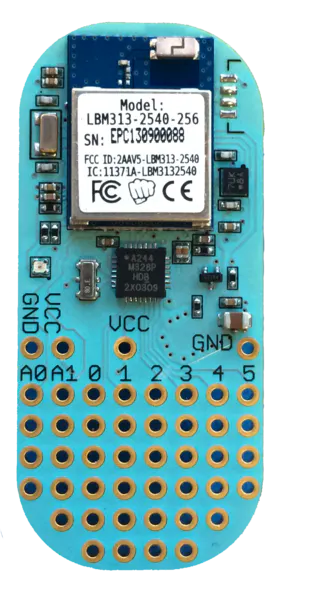# Move Rectangle In Processing Using LightBlue Bean

Move a rectangle in the screen using Processing 3, reading from the LightBlue Bean accelerometer. Data compiled as string and parsed later.

IntermediateProtip2 hours769## Things used in this project

### Hardware componentsPunch Through LightBlue Bean
×1

## Code

### AccelerometerToSerial.ino

Arduino
This is the file that runs in the LightBlue Bean. It will send the Accelerometer values written ax X,Y,Z to the serial port. Baud rate is 57600 and will need to be set in processing with the same number.
```void setup() {
Serial.begin (57600);
}

void loop() {
String stringToPrint = String();
stringToPrint = stringToPrint + acceleration.xAxis + "," + acceleration.yAxis + "," + acceleration.zAxis;
Serial.println(stringToPrint);
Bean.sleep(10);
}
```

### MoveRectLightBlueBean.pde

Java
After uploading AccelerometerToSerial.ino to the Bean and have it running, run this code in Processing to display a rect controlled by the bean's accelerometer.
```import processing.serial.*;

// Object to read from the serial port
Serial myPort;

// variables to store the accelerometer coordinates
int ax, ay, az;

// rectangle X position, Y position, Width and Height
int x, y, w, h;

void setup() {

// rectangle size (position will be defined dinamically)
w = 50;
h = 100;

// set canvas size. Because sensors read from -255 to 255, will need to add
// 255 to the value (to avoid negative positions). So values will be converted
// to 0 - 510, and considering the rectangle dimensions, this is the appropriate
// size for the canvas (510 + w, 510 + h)
size(560, 610);

// communication with the lighblue bean
myPort = new Serial(this, "/tmp/cu.Lightblue-Bean", 57600);

// rectangle color
fill (250, 200, 0);

}

void draw() {

while (myPort.available() > 0) {

// if it is not empty, process it
if (value != null && value.length() > 4) {
String[] sensors = value.split(",") ;
ax = Integer.parseInt(sensors.trim());
ay = Integer.parseInt(sensors.trim());
az = Integer.parseInt(sensors.trim());

// Sensor values goes from -255 to 255, so we need to add 255 and divide
// by 2 to convert into values from 0 to 255, and use for colors
int gray = (az + 255) / 2;

// change background to reproduce Z axis variation
background (gray, gray, gray);

// as we don't have negative position, need to add 255 (so it will
// be from 0 to 510)
x = ax + 255;
y = ay + 255;

// draw the rectangle
rect (x, y, w, h);

// A little pause to avoid lag
delay (50);

}

}
}
```

## Credits

### Paulo Amaral

1 project • 1 follower